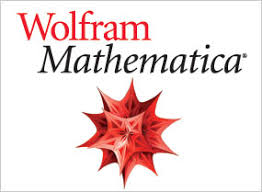-->

# Create Animation in MATHEMATICA

## Create Animation in MATHEMATICAMATHEMATICA is a modern technical computing system spanning most areas of technical computing — including neural networks, machine learning, image processing, geometry, data science, visualizations, and others. Many universities and scientist has been using MATHEMATICA now.  Now, Yoa will give you example to built an animation, that is making the particle fall (form a name) using Wolfram MATHEMATICA. By the way, you can buy Mathematica in this link "MATHEMATICA".

### Here is the syntax used,

Rasterize: rename "SRINK" in pixel form with pixel width of 160.
Binarize: Convert the name "SRINK" into an image that has a value of 0 and 1.
PixelValuePosition: displays the value of the pixel position of an image.
RandomReal: generates a Real value between 0 and 200.
Append: combine Real and pix values.
Graphics3D: create 3-dimensional graphics

### The result is as follows:

If you have any question about syntax above, feel free to write in comment.
Thanks/

Berlangganan update artikel terbaru via email: